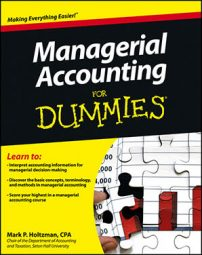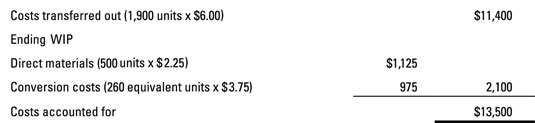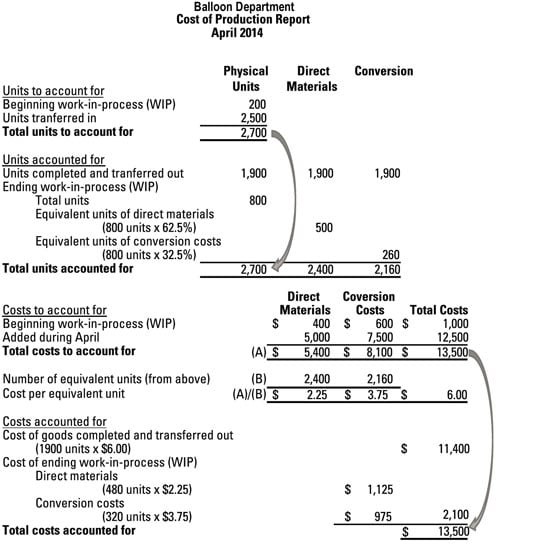##### Managerial Accounting For DummiesPart 4 of the cost of production report requires you to compute the costs accounted for, also called the cost reconciliation schedule. This schedule computes the cost of goods transferred out (based on the number of units transferred out times the cost per equivalent unit calculated in Part 3) plus the cost of WIP inventory remaining in the department at the end of the month.

Assume that Part 3 establishes that \$13,500 worth of costs went into the Balloon department. For your last and final trick, you have to prove that those \$13,500 worth of costs either remain in the Balloon department or have moved on to the next department. Just follow these steps:

1. Find the total cost of the units transferred out.

In this example, 1,900 units were transferred out of the Balloon department during April. Because these had a cost of \$6 per equivalent unit, the total cost of these units comes to \$11,400.

2. Compute the separate costs of WIP for direct materials and for conversion costs.

With respect to direct materials, the Balloon department had 500 equivalent units of WIP at the end of April. These equivalent units cost \$2.25 each, so the total cost of WIP at the end of April comes to \$1,125.

For conversion costs, the Balloon department had 260 equivalent units of WIP left over at the end of April. These equivalent units cost \$3.75 each, so their total cost comes to \$975. This amount, added to the cost of direct materials WIP above of \$1,125, gives you total WIP of \$2,100.

3. Add the total cost of units transferred out to the cost of WIP.

As explained in Step 1, the department transferred costs of \$11,400 to the next department. Furthermore, another \$2,100 worth of costs remain in the department with WIP. Therefore, total costs accounted for (the sum of these figures) amounts to \$13,500.Following is a complete cost of production report that accounts for all the Balloon department’s units and costs.